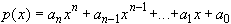#Interactive Real Analysis

Next | Previous | Glossary | Map

## 6.2. Continuous Functions

### Examples 6.2.6(a):

Every polynomial is continuous in R, and every rational function r(x) = p(x) / q(x) is continuous whenever q(x) # 0.
This follows by repeatedly applying the proposition on algebra on continuous functions:
• The identity function is continuous
• Multiplication of continuous functions yields continuous functions
• Hence, all monomials are continuous
• Sums and differences of continuous functions are continuous
• Hence, all polynomials are continuous.

To prove directly that a general polynomialis continuous is next to impossible. Taking the above detour makes the proof very easy. This is an example of a case where proving an abstract situation can be much simpler than proving a statement in a concrete situation.

The statement about rational functions being continuous now follows immediately from the fact that the division of two continuous functions yields another continuous function provided that the denominator is not zero.

Next | Previous | Glossary | Map